# Hypercomplex functions

Functionsof the hypercomplex variable(cf. Hypercomplex number) over the field of real numbers, i.e. a function on a finite-dimensional associative algebra. In a restricted sense, a hypercomplex function is a functionwith values in the same algebra, i.e. the function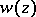may be represented as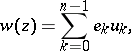where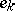,, is a basis of, while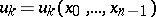,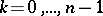, is a system ofreal functions inreal variables. The theory of hypercomplex functions has been most thoroughly studied for quaternion algebras(cf. Quaternion).

Analytic (regular) hypercomplex functions are generalizations in different directions of analytic functions of one complex variable. The concepts of an analytic hypercomplex function differ, on account of the fact that the definitions of analyticity in arbitrary algebras need not be equivalent.

In modern studies principal stress is laid on regular hypercomplex functions analytic according to Fueter, or-analytic hypercomplex functions, . A hypercomplex function is said to be right regular at a point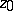if the differential equation (Fueter's condition)whereis the partial derivative of the function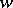with respect to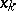, is satisfied at that point; all derivatives are assumed to be continuous. A functionis said to be a left-regular hypercomplex function ifIn the case of a non-commutative algebrathese concepts are not equivalent. The sum and the difference of right-regular hypercomplex functions are right regular, but this is not true for their product or quotient. Powers of the variableare not right-regular. There exist Taylor and Laurent series for specially constructed analogues of powers. Fueter's condition is equivalent to the vanishing of the differential of the hypercomplex differential form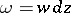,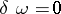(for left-regular hypercomplex functions — of the form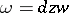); hence a specific integral theorem is obtained.

A hypercomplex function analytic according to Scheffers  at a pointfor the case of a commutative algebrais a hypercomplex function for which the differential at that point may be written aswhere the derivative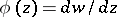is independent of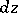. In the case of a commutative algebrathis condition is equivalent to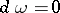, and the integral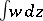is independent of the path. Hypercomplex functions that are analytic according to Scheffers are-regular if and only ifA hypercomplex functionis said to be analytic according to Hausdorff  at a pointif its differential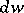is a linear function of, i.e. if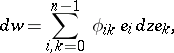whereare real functions in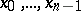. In this case the analogues of power series are easier to construct, but the value of the integral depends on the path. For a commutative algebraHausdorff's and Scheffers' definitions are equivalent.

How to Cite This Entry:
Hypercomplex functions. E.D. Solomentsev (originator), Encyclopedia of Mathematics. URL: http://encyclopediaofmath.org/index.php?title=Hypercomplex_functions&oldid=13128
This text originally appeared in Encyclopedia of Mathematics - ISBN 1402006098